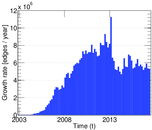# Wikipedia edits (ru)

This is the bipartite edit network of the Russian Wikipedia. It contains users and pages from the Russian Wikipedia, connected by edit events. Each edge represents an edit. The dataset includes the timestamp of each edit.

 Code `ru` Internal name `edit-ruwiki` Name Wikipedia edits (ru) Data source http://dumps.wikimedia.org/ AvailabilityDataset is available for download Consistency checkDataset passed all tests Category Authorship network Dataset timestamp 2017-10-20 Node meaning User, article Edge meaning Edit Network formatBipartite, undirected Edge typeUnweighted, multiple edges Temporal dataEdges are annotated with timestamps

## Statistics

 Size n = 5,746,517 Left size n1 = 414,198 Right size n2 = 5,332,319 Volume m = 65,690,151 Unique edge count m̿ = 27,686,279 Wedge count s = 1,241,988,684,252 Maximum degree dmax = 1,172,770 Maximum left degree d1max = 1,172,770 Maximum right degree d2max = 210,499 Average degree d = 22.862 6 Average left degree d1 = 158.596 Average right degree d2 = 12.319 2 Fill p = 1.253 55 × 10−5 Average edge multiplicity m̃ = 2.372 66 Size of LCC N = 5,625,375 Diameter δ = 13 50-Percentile effective diameter δ0.5 = 3.484 77 90-Percentile effective diameter δ0.9 = 3.963 35 Median distance δM = 4 Mean distance δm = 3.933 09 Balanced inequality ratio P = 0.129 679 Left balanced inequality ratio P1 = 0.046 996 7 Right balanced inequality ratio P2 = 0.172 766 Degree assortativity ρ = −0.073 332 1 Degree assortativity p-value pρ = 0.000 00 Controllability C = 5,075,008 Relative controllability Cr = 0.893 344

## Plots

### Degree distribution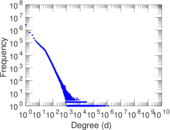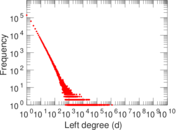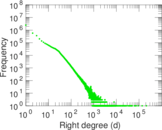### Cumulative degree distribution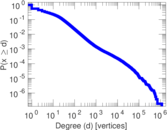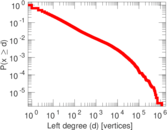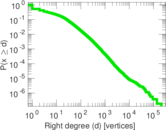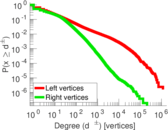### Spectral distribution of the adjacency matrix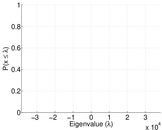### Spectral distribution of the normalized adjacency matrix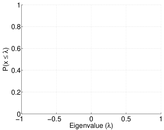### Spectral distribution of the Laplacian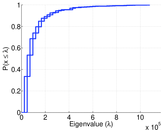### Spectral graph drawing based on the adjacency matrix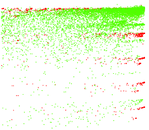### Spectral graph drawing based on the normalized adjacency matrix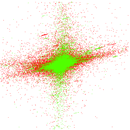### Hop distribution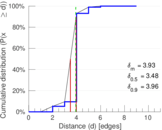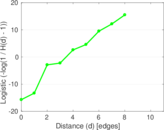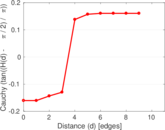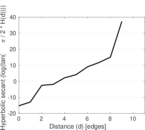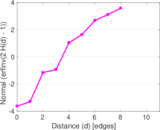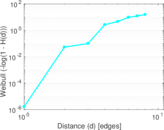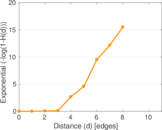### Edge weight/multiplicity distribution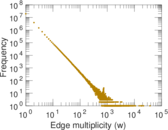### Temporal distribution